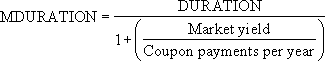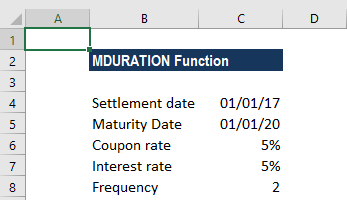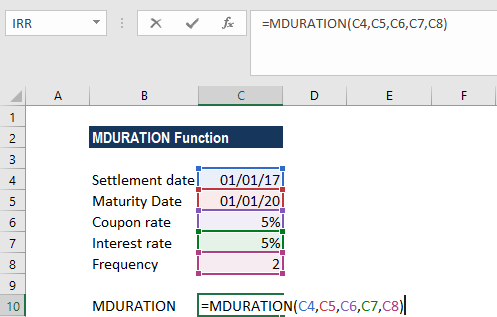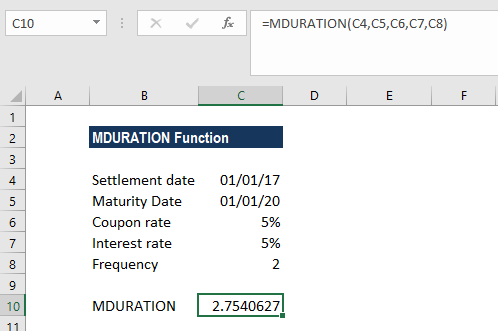# MDURATION Function

Calculates the duration of a security using the Modified Macauley method

## What is the MDURATION Function?

The MDURATION Function is categorized under Excel Financial functions. It will calculate the duration of a security using the Modified Macauley method.

In financial analysis, we often evaluate a bond and develop a strategy that will match the duration of assets and liabilities. This is done to minimize the impact of interest rates on the net worth of a business. The MDURATION function helps us formulate a similar strategy.

The Modified Duration is defined as follows:MDURATION is very similar to the DURATION function. The MDURATION function returns the annual duration of a security with periodic interest payments, but it calculates the duration if the yield were to increase 1%.

The Macaulay duration is calculated for an assumed par value of \$100. Both duration and modified duration allow bonds of different maturities and coupon rates to be compared directly. It can be useful to use DURATION in conjunction with MDURATION.

### Formula

=MDURATION(settlement, maturity, coupon, yld, frequency, [basis])

The MDURATION function uses the following arguments:

1. Settlement (required argument) – This is the settlement date of the security after the issue date when the security is traded to the buyer.
2. Maturity (required argument) – This is the maturity date of the security or the date when the security expires.
3. Coupon (required argument) – The annual coupon rate.
4. Yld (required argument) – This is the annual yield of the security.
5. Frequency (required argument) – This is the number of coupon payments per year. The frequency is 1 for annual payments, 2 for semi-annual, and 4 for quarterly payments.
6. Basis (optional argument) – This is the type of day count basis to use.

BasisDay Count basis
0 or omittedUS(NASD) 30/360
1Actual/actual
2Actual/360
3Actual/365
4European 30/360

### How to use the MDURATION Function in Excel?

To understand the uses of the MDURATION function, let’s consider an example:

#### Example

Let’s assume that a bond is priced at \$10,000, pays a 5% coupon, and matures in three years. The interest rate is 5%. The bond pays the coupon twice a year and pays the principal on the final payment.The formula to use will be:We get the result below:Here, we omitted the basis argument, so the function used the default value 0 (denoting the US (NADS) 30/360 day count basis).

### A few notes about the MDURATION Function

1. Remember that Excel stores date in sequential serial numbers so they can be used in calculations. By default, January 1, 1900 is serial number 1, and January 1, 2017 is serial number 42736 because it is 42,736 days after January 1, 1900.
2. #NUM! error – Occurs when:
1. The given settlement date is greater than or less than the maturity date.
2. If coupon, yld, frequency or basis arguments given are invalid numbers.
3. The MDURATION function will truncate the decimal in settlement, maturity, frequency, or basis to integers.
4. The date arguments should be supplied to the function as either:
1. References to cells containing dates; or
2. Dates returned from formulas.
5. #VALUE! error – Occurs when:
1. Any of the given argument is non-numeric.
2. Any of the given settlement or maturity dates are invalid MS Excel dates.

Thanks for reading CFI’s guide to important Excel functions! By taking the time to learn and master these functions, you’ll significantly speed up your financial analysis. To learn more, check out these additional CFI resources:

• Excel Functions for Finance